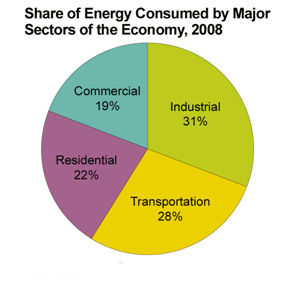Wiki
Home » Blog » Wiki » Determine coefficient of grounding

# Determine coefficient of grounding

Determination of required grounding impedance is based on determination of coefficient of grounding which represents ratio of maximum phase voltage at phases which aren't exposed by fault and line voltage of power network:

kuz=(1/(sqrt(3)))*max{|e(-j*2*π/3)+(1-z)/(2+z)|; |e(+j*2*π/3)+(1-z)/(2+z)|}
z=Z0e/Zde

where are:

kuz-coefficient of grounding,
z-ratio of equivalent zero sequence impedance viewed from angle of place of fault and equivalent direct sequence impedance viewed from angle of place of fault,
Z0e-equivalent zero sequence impedance viewed from angle of place of fault,
Zde-equivalent direct sequence impedance viewed from angle of place of fault.

So, after this explanation, you can get next conclusions:
if kuz=1 then power network is ungrounded because Z0e→∞, which is a consequence of existing more (auto) transformers with ungrounded neutral point than (auto) transformers with grounded neutral point (when kuz=1 then there aren't (auto) transformers with grounded neutral point),
if kuz≤0,8 then power network is grounded because Z0e=Zde, which is a consequence of of existing more (auto) transformers with grounded neutral point than (auto) transformers with ungrounded neutral point.

Fault current in grounded power networks is higher than fault current in ungrounded power networks. By other side, in case of ungrounded power networks we have overvoltages at phases which aren't exposed by fault, so insulation of this conductors could be seriously damaged or in best case it could become older in shorter time than it is provided by design what is the main reason for grounding of power networks.
Coefficient of grounding is very important in aspect of selecting of insulation of lighting arresters and breaking power of breakers, because of two next reasons:
1. in grounded power networks insulation level is lower than insulation level in ungrounded power networks,
2. in grounded power networks value of short circuit current is higher than value of short circuit current in ungrounded power networks.

Calculate (2 - 4) =

You may also like:

AC Motors - Variable torque: AC motors have a speed torque characteristic that varies as the square of the speed. AC Motors - Constant torque: These ac motors can develop the same torque at each speed, thus ...
Two electrical motors that design for altitude <1000 m but now this two electrical motor have installed on altitude 1880 m and this electrical motors become very hot. The electrical machines power is ...
The key to optimizing the system operation is communication and information sharing through the entire system equipment. With the reduced cost of variable frequency drives and Building Automation Systems, ...
Due to variable frequency drive can adapt to various requirements in production process, especially in the industrial automation control applications, AC variable frequency control technology has risen to be ...
If you are interested in power electronics design at the board or system level, I would recommend LTspice (note the correct spelling) by far above all the others. In addition to being superb for IC design ...Gozuk Blog: all about electric motor control & drives industries development in energy saving applications.

Featured

Like pumps, fans consume significant electrical energy while serving several applications. In many plants, the VFDs (variable ...A frequency inverter controls AC motor speed. The frequency inverter converts the fixed supply frequency (60 Hz) to a ... Motor starter (also known as soft starter, motor soft starter) is a electronic device integrates soft start, soft stop, ... Soft starter allows the output voltage decreases gradually to achieve soft stop, in order to protect the equipment. Such as the ... Soft Starter reduces electric motor starting current to 2-4 times during motor start up, reduces the impact to power grid during ...

In Discussion